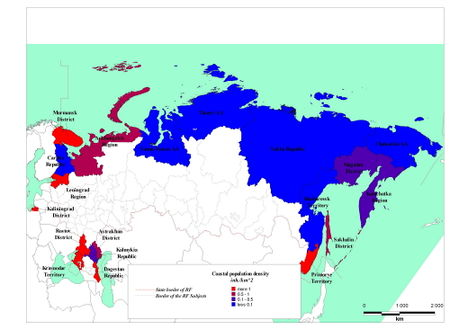# Evaluation of demographic marine-economics potential: the Russian example

## Methodological aspects

The method described in Comprehensive assessment of coastal regions: the Russian example requires a large informational base, and besides, the ingredients represent a rather hard problem with ambiguous algorithms of parameterization and estimation. However in some cases it is possible to simplify the task, by applying methods of the preliminary analysis (so-called express analysis or the express-estimation), which allows identification of the basic priority regions.

The basic difficulty in usage of this method consists in search and substantiation of choice of adequate indicators. For research of a social and economic significance and sustainability of development of coastal regions, an adequate parameter can be the relative population in human coastal settlements. As indicator for the common analysis of demographic aspects of coastal regions it is possible to use a demographic marine-economics potential ($DMEP$).

Considering the characteristics defining the given parameter for conditions, it is possible to present its expression as aggregate of following numerical complexes:

$~DMEP=f(\large\frac {P_R}{S_R}, \frac {P_R}{L}, \frac {n}{H}, \frac {D_R}{D}\normalsize)$,

where $P_R$ = population of coastal areas of region, thousand; $S_R$ = the total area of coastal areas of region [$10^3 km^2$], $L$ = extent of shore line of coastal region [$km$]; $H$ = the weighted-mean distance of living of inhabitants of human settlements up to shore line [$km$]; $n$ = quantity of cities, towns and other urban settlements, and also administrative centers of local municipalities of coastal areas of the region; $D$ = population density of coastal region, in general [$inh . km^{-2}$]; $D_R$ = population density of coastal areas of the region [$inh. km^{-2}$].

As human settlements one may understand all cities, towns and other urban settlements and also administrative centers of coastal region of lower level of governance (i.e. local municipalities, as lower level of governance of province, for example).

Proceeding from kinds of associations (direct or inverse) of required function and parameters, the functional expression for $DMEP$ parameter can be cast in the form:

$~DMEP = \sqrt{\large\frac {P_R^2 \cdot n}{S_R \cdot LH}} \cdot \frac {D_R}{D}\normalsize = \sqrt{\large\frac {n}{S_R \cdot LH}} \cdot P_R \cdot \frac {D_R}{D}\normalsize$,

Thus the value of the weighted-mean distance $H$ is as:

$~H = \large\frac {\sum^{n}_{i=1}{P_i \cdot H_i}} {\sum^{n}_{i=1}{P_i}}\normalsize$,

where $P_i$ = population of human settlement [$10^3$]; $H_i$ = distance from human settlement up to shore line [$km$].

Total $DMEP$ value is a quantity with dimensional [$inh.km^{-2}$], i.e. dimension of population density. Thus, the given parameter reflects a degree of possible security of considered coastal region by labor forces for realization of marine activity, which in the following is designated as coastal population density ($CPD$).

## Opportunity of application of indicator techniques for the coastal zones on the regional level

For the analysis of coastal regions of the Russian Federation we shall enter following assumptions.

First, as coastal regions of the Russian Federation we shall understand coastal Subjects of the Russian Federation.

Secondly, for more correct analysis we shall consider the following aggregates of Subjects as one region:

• The Arkhangelsk region, as aggregate of the Arkhangelsk District and Nenets Autonomous Area.
• The Leningrad region, as aggregate of Leningrad District and St. Petersburg.

Thirdly, Taimyr (Dolgano-Nenets) Autonomous Area is considered as separate region, not as the constituent of Krasnoyarsk Territory which he is since January, 1st, 2007.

So, there are 19 coastal regions of the Russian Federation.

## General analysis of demographic aspects of coastal regions of the Russian Federation

Parameters and values of coastal population density are showed in table 1 and in figure 1.Table 1. Distribution of values of coastal population density ($CPD$) on regions of the Russian FederationFigure 1. Distribution of coastal population density ($CPD$) values on regions of the Russian Federation

By estimating coastal population density values the ranking of following groups of regions is possible:

• Regions with high values of coastal population density (Murmansk District, Primorye Territory, Dagestan Republic, Krasnodar Territory, Rostov District, Kaliningrad District and Leningrad Region), for which the value of coastal population density more than $1 \, inh.km^{-2}$ (more than 0 on a logarithmic scale);
• Regions with significant values of coastal population density (Arkhangelsk Region, Sakhalin District and Astrakhan District), for which the values of coastal population density more than $0.3 \, inh.km^{-2}$ (more than -1 on a logarithmic scale);
• Regions with negligible values of coastal population density (Magadan District, Kamchatka Region and Kalmykia Republic), for which the values of coastal population density more than $0.1 \, inh.km^{-2}$ (more -2 on a logarithmic scale);
• Regions with low values of coastal population density (Kareliya Republic, Yamal-Nenets Autonomous Area, Taimyr (Dolgano-Nenets) Autonomous Area, Sakha Republic, Chukotski Autonomous Area and Khabarovsk Territory), for which the values of coastal population density less than $0.1 \, inh.km^{-2}$ (less -2 on a logarithmic scale).

As a result, it is possible to conclude that the best perspective for regional development of marine activity from the demographic point of view correspond to regions with high and significant values of coastal population density, and partially regions with negligible values of coastal population density for which the development of the concentrated branches of marine activity is possible. This means that only 13 coastal regions of the Russian Federation have really possibilities to develop a marine activity policy. Regions with low coastal population density have low perspectives for development of marine activity, firstly because of their demographic weakness.